• 通过代码，了解卷积和池化计算过程 import cv2 as cv import numpy as np from scipy import misc import matplotlib.pyplot as plt i =misc.ascent()# 获得一张图片 i_transformed = np.copy(i) size_x = i_...
通过代码，了解卷积和池化的计算过程
import cv2 as cv
import numpy as np
from scipy import misc
import matplotlib.pyplot as plt

i =misc.ascent()# 获得一张图片

i_transformed = np.copy(i)
size_x = i_transformed.shape#
size_y= i_transformed.shape#

#设置一个过滤器
filter = [ [-1, -2, -1], [0, 0, 0], [1, 2, 1]]
# 如果过滤器中的所有数字加起来不是0或1，那么就应该设置权重，让过滤器的总和为0或1。
# 例如，如果过滤器是1，1，1，1，2，1，1，1，1。
# 它们加起来是10，所以可以设置0.1的权重，使它标准化。
weight  = 1
'''
接下来让我们创建一个卷积，扫描整个图像，留出1个像素的边框，并将当前像素的每个邻居乘以过滤器中定义的值。

即当前像素上方和左侧的邻域将乘以滤镜中左上方的项，等等。然后我们再将结果乘以权重，然后确保结果在0-255的范围内。

最后我们将把新的值加载到转换后的图像中'''
for x in range(1,size_x-1):
for y in range(1,size_y-1):
convolution =0.0

for x in range(1, size_x - 1):
for y in range(1, size_y - 1):
convolution = 0.0
convolution = convolution + (i[x - 1, y - 1] * filter)
convolution = convolution + (i[x, y - 1] * filter)
convolution = convolution + (i[x + 1, y - 1] * filter)
convolution = convolution + (i[x - 1, y] * filter)
convolution = convolution + (i[x, y] * filter)
convolution = convolution + (i[x + 1, y] * filter)
convolution = convolution + (i[x - 1, y + 1] * filter)
convolution = convolution + (i[x, y + 1] * filter)
convolution = convolution + (i[x + 1, y + 1] * filter)
convolution = convolution * weight
if (convolution < 0):
convolution = 0
if (convolution > 255):
convolution = 255
i_transformed[x, y] = convolution
#将一个3x3的像素块经过运算转化为1x1的像素点

plt.gray()
plt.grid(False)
plt.imshow(i_transformed)
#plt.axis('off')
plt.show()
plt.imshow(i_transformed)
plt.show(i_transformed)

'''
这段代码将显示一个（2，2）池化后的效果。它的想法是用2x2尺寸的矩阵在图像上扫描，查看像素和它的右方、下方和右下方的近邻像素。取其中最大的一个，并将其加载到新图像中。这样，新的图像将是旧图像的1/4大小--通过这个过程，X和Y上的尺寸减半。你会发现，尽管进行了这样的压缩，图像特征还是得到了保留。'''

new_x = int(size_x / 2)
new_y = int(size_y / 2)
newImage = np.zeros((new_x, new_y))
for x in range(0, size_x, 2):
for y in range(0, size_y, 2):
pixels = []
pixels.append(i_transformed[x, y])
pixels.append(i_transformed[x + 1, y])
pixels.append(i_transformed[x, y + 1])
pixels.append(i_transformed[x + 1, y + 1])
newImage[int(x / 2), int(y / 2)] = max(pixels)

# Plot the image. Note the size of the axes -- now 256 pixels instead of 512
plt.gray()
plt.grid(False)
plt.imshow(newImage)
# plt.axis('off')
plt.show()




展开全文过滤器
• 最近在做实验是，发现看代码可以增加自己的认识，就最近对卷积的操作的总结 方便今后的查阅， 在卷积神经网络中，卷积算是一...下图是在一个卷积层中将卷积操作展开的具体操作过程，他里面按照卷积核的大小取数据然后展
最近在做实验是，发现看代码可以增加自己的认识，就最近对卷积的操作的总结
方便今后的查阅，也矫正之前的理解偏差。
在卷积神经网络中，卷积算是一个必不可少的操作，
下图是一个简单的各层的关系。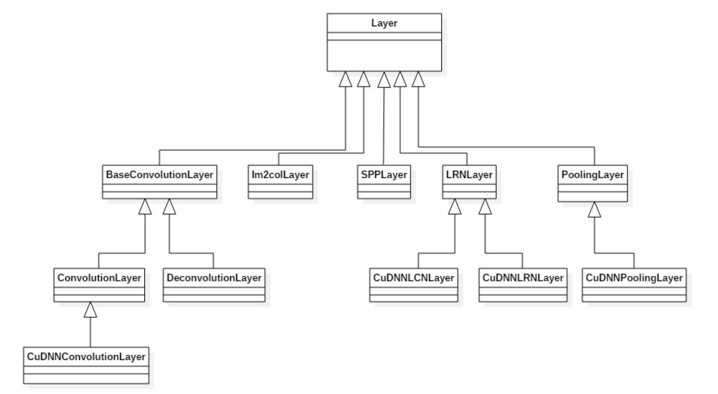可以看出一个很好的扩展的关系，下面是整个卷积的大概的过程
图中上半部分是传统的卷积的操作，下图是一个矩阵的相乘的操作。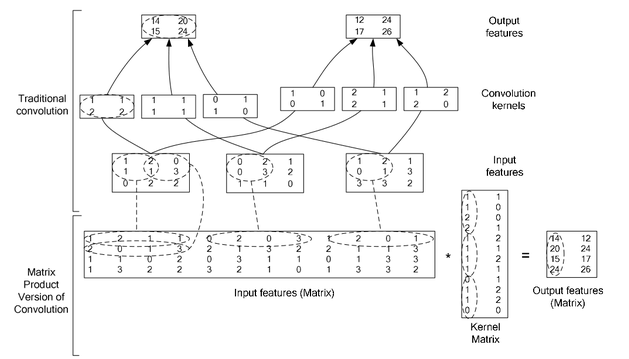下图是在一个卷积层中将卷积操作展开的具体操作过程，他里面按照卷积核的大小取数据然后展开，在同一张图里的不同卷积核选取的逐行摆放，不同N的话，就在同一行后面继续拼接，不同个可以是多个通道，但是需要注意的是同一行里面每一段都应该对应的是原图中中一个位置的卷积窗口。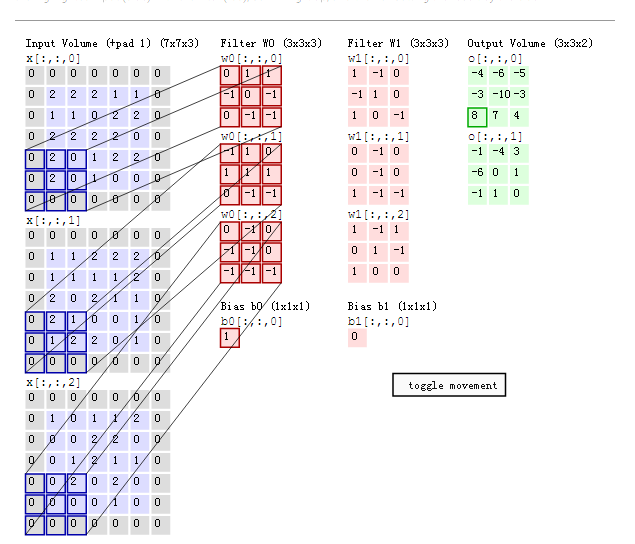从上图可以清晰的看到卷积的计算过程是
将一个三通道的RGB的图像拆分成三张单通道的图像，然后针对每一个通道都有一个k个卷积核，进行卷积操作，从图中可以清晰看到每一个通道的对应的K个卷积和都是不同的，但是在计算完成之后，在将对应的卷积的结果进行叠加，即可，这样就得到整个卷积层的特征图像了。下一步就是其他的操作。

下图是在一个卷积层中将卷积操作展开的具体操作过程，他里面按照卷积核的大小取数据然后展开，在同一张图里的不同卷积核选取的逐行摆放，不同N的话，就在同一行后面继续拼接，不同个可以是多个通道，但是需要注意的是同一行里面每一段都应该对应的是原图中中一个位置的卷积窗口。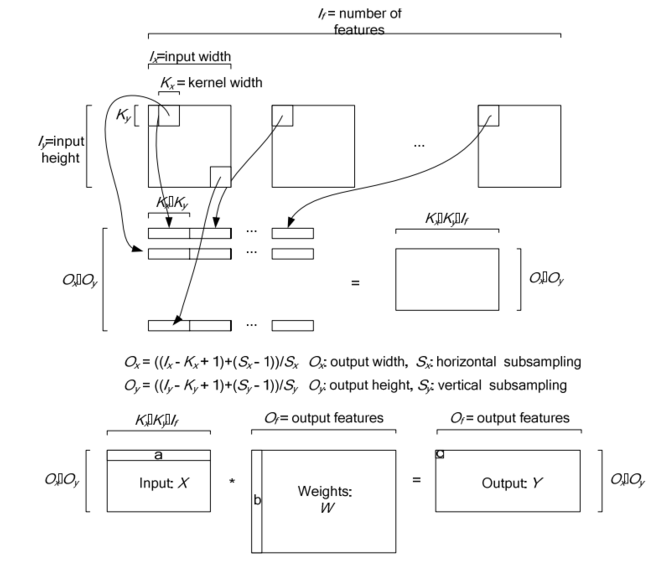对于卷积层中的卷积操作，还有一个group的概念要说明一下，groups是代表filter 组的个数。引入gruop主要是为了选择性的连接卷基层的输入端和输出端的channels，否则参数会太多。每一个group 和1/ group的input 通道和
1/group 的output通道进行卷积操作。比如有4个input，
8个output，那么1-4属于第一组，5-8属于第二个gruop

展开全文• 对于卷积神经网络中，卷积操作可能是最常见操作，具体原理可以去学习一下Andred NG课程，建议搞计算机视觉方向都去刷一波，具体过程如图1所示： 图1 'VALID'方式卷积操作过程 其实就是卷积核与图像待操作...
对于卷积神经网络中，卷积操作可能是最常见操作，具体原理可以去学习一下Andred NG的课程，建议搞计算机视觉方向的都去刷一波，具体过程如图1所示：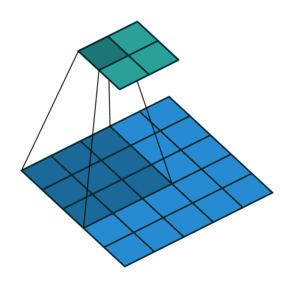图1 'VALID'方式卷积操作过程

其实就是卷积核与图像待操作区域进行乘加操作，常见的卷积操作有两种形式，第一种是'VALID'的方式，如图1所示，第二种是'SAME'的方式，区别在于'SAME'方式会对输入进行填充，以保证卷积操作之后，输出的size和输入的size一致。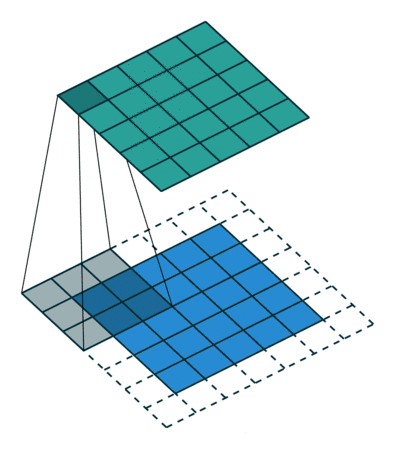图2 'SAME'方式卷积操作过程

1、padding

先说一下填充padding，padding就是在原始图像四周填充0，对应于图2中虚线部分，使用tvm实现，代码如下：

def padding(X, ph, pw):
assert len(X.shape) >= 2
nh, nw = X.shape[-2], X.shape[-1]
return tvm.compute(
(*X.shape[0:-2], nh + ph * 2, nw + pw * 2),
lambda *i: tvm.if_then_else(
tvm.any(i[-2] < ph, i[-2] >= nh + ph, i[-1] < pw, i[-1] >= nw + pw),
0, X[i[:-2] + (i[-2] - ph, i[-1] - pw)]
), name = 'PaddedX'
)

2、输出feature map尺寸计算

对于 输入size为n， 卷积核size为k， 填充size为p，卷积操作步长size为s，输出大小为：

对应代码如下：

def conv_out_size(n, k, p, s):
return (n - k + 2 * p) // s + 1

3、卷积操作

就是将卷积核与要操作的图像块进行乘加操作，对应于tvm代码为：

def conv(oc, ic, nh, nw, kh, kw, ph=0, pw=0, sh=1, sw=1):
# reduction axes
ric = tvm.reduce_axis((0, ic), name='ric')
rkh = tvm.reduce_axis((0, kh), name='rkh')
rkw = tvm.reduce_axis((0, kw), name='rkw')

# output height and width
oh = conv_out_size(nh, kh, ph, sh)
ow = conv_out_size(nw, kw, pw, sw)

# pad x and then conpute y
X = tvm.placeholder((ic, nh, nw), name='x')
K = tvm.placeholder((oc, ic, kh, kw), name='k')
# 对输入填充
PaddedX = padding(X, ph, pw) if ph * pw != 0 else X
Y = tvm.compute(
(oc, oh, ow),
lambda c, i, j: tvm.sum(
PaddedX[ric, i * sh + rkh, j * sw + rkw] * K[c, ric, rkh, rkw],
axis=[ric, rkh, rkw]
), name='Y'
)

return X, K, Y, PaddedX

最后，看一下实际生成的伪代码：

import tvm
import numpy as np
import mxnet as mx

def padding(X, ph, pw):
assert len(X.shape) >= 2
nh, nw = X.shape[-2], X.shape[-1]
return tvm.compute(
(*X.shape[0:-2], nh + ph * 2, nw + pw * 2),
lambda *i: tvm.if_then_else(
tvm.any(i[-2] < ph, i[-2] >= nh + ph, i[-1] < pw, i[-1] >= nw + pw),
0, X[i[:-2] + (i[-2] - ph, i[-1] - pw)]
), name = 'PaddedX'
)

# 输入size：n
# 卷积核size：k
# 填充size：p
# 步长size：s
def conv_out_size(n, k, p, s):
return (n - k + 2 * p) // s + 1

def conv(oc, ic, nh, nw, kh, kw, ph=0, pw=0, sh=1, sw=1):
# reduction axes
ric = tvm.reduce_axis((0, ic), name='ric')
rkh = tvm.reduce_axis((0, kh), name='rkh')
rkw = tvm.reduce_axis((0, kw), name='rkw')

# output height and width
oh = conv_out_size(nh, kh, ph, sh)
ow = conv_out_size(nw, kw, pw, sw)

# pad x and then conpute y
X = tvm.placeholder((ic, nh, nw), name='x')
K = tvm.placeholder((oc, ic, kh, kw), name='k')
# 对输入填充
PaddedX = padding(X, ph, pw) if ph * pw != 0 else X
Y = tvm.compute(
(oc, oh, ow),
lambda c, i, j: tvm.sum(
PaddedX[ric, i * sh + rkh, j * sw + rkw] * K[c, ric, rkh, rkw],
axis=[ric, rkh, rkw]
), name='Y'
)

return X, K, Y, PaddedX

def get_conv_data(oc, ic, n, k, p=0, s=1, constructor=None):
np.random.seed(0)
data = np.random.normal(size=(ic, n, n)).astype('float32')
weight = np.random.normal(size=(oc, ic, k, k)).astype('float32')
on = conv_out_size(n, k, p, s)
out = np.empty((oc, on, on), dtype='float32')
if constructor:
data, weight, out = (constructor(x) for x in [data, weight, out])

return data, weight, out

oc, ic, n, k, p, s = 4, 6, 12, 3, 1, 1
X, K, Y, _ = conv(oc, ic, n, n, k, k, p, p, s, s)
sch = tvm.create_schedule(Y.op)
mod = tvm.build(sch, [X, K, Y])
print(tvm.lower(sch, [X, K, Y], simple_mode=True))

data, weight, out = get_conv_data(oc, ic, n, k, p, s, tvm.nd.array)
mod(data, weight, out)

def get_conv_data_mxnet(oc, ic, n, k, p, s, ctx='cpu'):
ctx = getattr(mx, ctx)()
data, weight, out = get_conv_data(oc, ic, n, k, p, s,
lambda x: mx.nd.array(x, ctx=ctx))
data, out = data.expand_dims(axis=0), out.expand_dims(axis=0)
bias = mx.nd.zeros(out.shape, ctx=ctx)
return data, weight, bias, out

def conv_mxnet(data, weight, bias, out, k, p, s):
mx.nd.Convolution(data, weight, bias, kernel=(k, k), stride=(s, s),
pad=(p, p), num_filter=out.shape, out=out)

data, weight, bias, out_mx = get_conv_data_mxnet(oc, ic, n, k, p, s)
conv_mxnet(data, weight, bias, out_mx, k, p, s)
np.testing.assert_allclose(out_mx.asnumpy(), out.asnumpy(), atol=1e-5)


输出为：

// attr [PaddedX] storage_scope = "global"
allocate PaddedX[float32 * 1176]
produce PaddedX {
for (i0, 0, 6) {
for (i1, 0, 14) {
for (i2, 0, 14) {
PaddedX[(((i0*196) + (i1*14)) + i2)] = tvm_if_then_else(((((i1 < 1) |
| (13 <= i1)) || (i2 < 1)) || (13 <= i2)), 0f, x[((((i0*144) + (i1*12)) + i2) - 13)])      }
}
}
}
produce Y {
for (c, 0, 4) {
for (i, 0, 12) {
for (j, 0, 12) {
Y[(((c*144) + (i*12)) + j)] = 0f
for (ric, 0, 6) {
for (rkh, 0, 3) {
for (rkw, 0, 3) {
Y[(((c*144) + (i*12)) + j)] = (Y[(((c*144) + (i*12)) + j)] + (P
addedX[(((((ric*196) + (i*14)) + (rkh*14)) + j) + rkw)]*k[((((c*54) + (ric*9)) + (rkh*3)) + rkw)]))            }
}
}
}
}
}
}


参考资料：

 https://blog.csdn.net/kingroc/article/details/88192878

 http://tvm.d2l.ai.s3-website-us-west-2.amazonaws.com/

展开全文• 下图是卷积操作： 下图是转置卷积（反卷积）： 空洞卷积（扩张卷积）： 空洞卷积也有自己缺点，需要设计一些精巧组合来保证视野域能覆盖比较紧密。具体参考下面讨论： 知乎讨论《如何理解空洞卷积...
github上有一个项目用于演示各种卷积的动态过程：https://github.com/vdumoulin/conv_arithmetic
下图是卷积操作：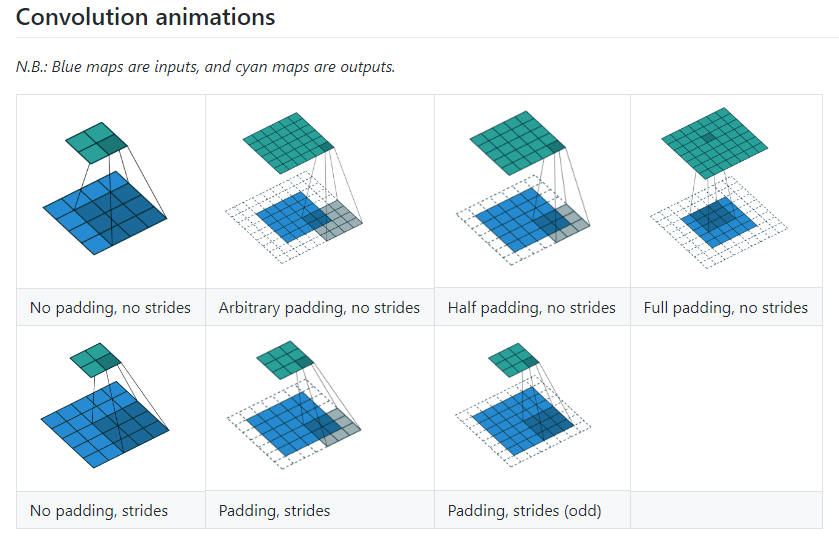下图是转置卷积（反卷积）：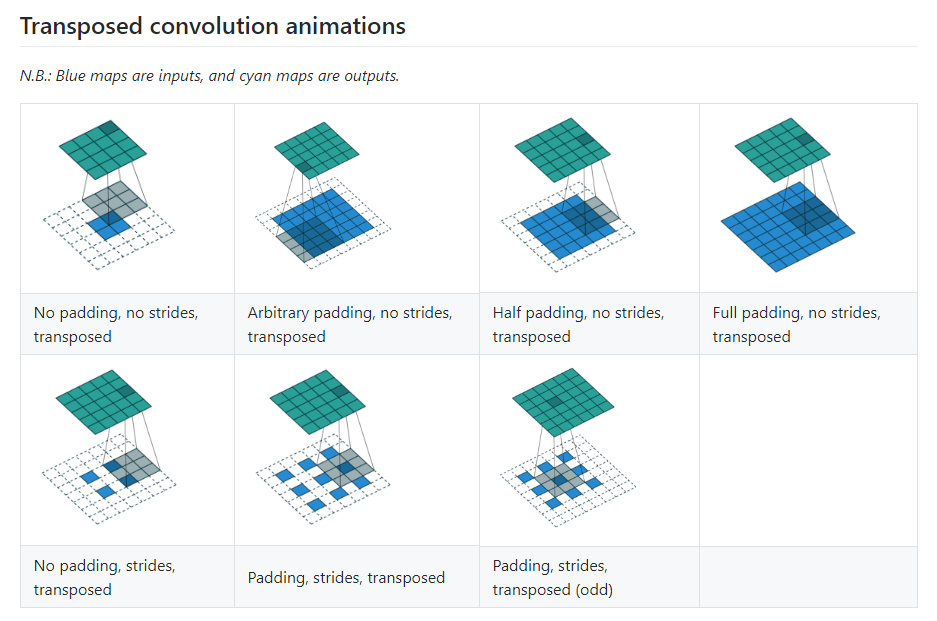空洞卷积（扩张卷积）：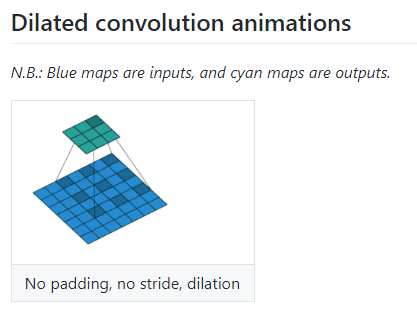空洞卷积也有自己的缺点，需要设计一些精巧的组合来保证视野域能覆盖的比较紧密。具体参考下面的讨论：
知乎讨论《如何理解空洞卷积（dilated convolution）？》：https://www.zhihu.com/question/54149221
参考资料
上面的截图是根据这篇论文的内容制作的，内容很详细，值得参考：
《A guide to convolution arithmetic for deep
learning》：https://arxiv.org/pdf/1603.07285.pdf
下面的文章讲解也很详细：
万字长文带你看尽深度学习中的各种卷积网络（上篇）
万字长文带你看尽深度学习中的各种卷积网络（下篇）


展开全文• 在卷积神经网络中，卷积算是一个必不可少的操作， ...下图是在一个卷积层中将卷积操作展开的具体操作过程，他里面按照卷积核的大小取数据然后展开，在同一张图里的不同卷积核选取的逐行摆放，不同N的话，就在同一行
• 具体过程如下： （1）首先将卷积与感知野乘积转换一下，将4*4的卷积核转换为下面矩阵，然后4*4原始数据转换为16*1向量，这样子下面矩阵一共有四行对应了四次卷积运算，0则表示在实际卷积过程中，卷积核与...
• 参考： ...1 普通卷积 CNN中普通卷积是三维卷积，每个卷积通道数和输入数据通道数相同，卷积个数为输出数据...具体的计算过程为在各通道进行卷积核和对应感受野输入数据对应位置相乘再相加操作，得到一个...
• 卷积操作是使用一个二维卷积核在在批处理图片中进行扫描，具体的操作是在每一张图片上采用合适窗口大小在图片每一个通道上进行扫描。 权衡因素：在不同通道和不同卷积核之间进行权衡 在tensorflow中...
• 卷积本身执行过程是通过在特征图上滑动卷积核来完成。但是在Caffe底层实现是通过矩阵相乘方式一步完成卷积的操作。 假设输入图像及卷积和大小如下图所示： 在Caffe实现中，它分别将特征图和卷积转换为...
• 卷积步长是构建卷积神经网络一个基本操作 前几篇博客都是步长为1的卷积神经网络例子，现在以步长为2例子来说明步长不为1情况。 下面是一个7x7灰度图像矩阵，以及一个3x3过滤器，卷积运算之后...
• ## 反卷积原理

千次阅读 2018-05-13 15:17:20
这里千万不要当成反卷积操作可以复原卷积操作的输入值，反卷积并没有那个功能，它仅仅是将卷积变换过程中的步骤反向变换一次而已，通过将卷积核转置，与卷积后的结果再做一遍卷积，所以它还有个名字叫转置卷积。...
• 卷积神经网络的具体操作卷积过程代码实现4.池化层(Pooling Sampling)下采样上采样5.批处理规范(Batch Norm)Image NormalizationBatch Normalization 1.关于图像的基础知识 灰度图 这里以MINST数据集为例，对于一个...人工智能 神经网络
• 图像卷积操作的应用没有严格的数学推导，即没有数学推导表明每一层究竟表示什么。 为了了解卷积神经网络中每一层与原有图像的对应关系， 文章Visualizing and Understanding Convolutional Networks通过反向卷积...
• 主流框架包括caffe、tf、darknet实现卷积的时候不是直接进行卷积计算，而是往往采用im2col+gemm方式来进行加速，加速思路是“空间换时间”提高读取数据过程cache命中。本文主要利用ARMNEON汇编指令来加速...算法 深度学习 neon arm
• 为了接下来要讲的图像的滤波和边缘处理，这里介绍详细介绍下一维、二维卷积操作的过程。这里我不一开始就拿出公式，从其物理意义入手可能会更好理解，其中可能会涉及一些概念，我没具体展开的、想要深入了解的朋友请...
• 最近在读‘MULTI-SCALE CONTEXT AGGREGATION BY DILATED CONVOLUTIONS’这篇论文，里面提到了DILATED ...上图是具体的计算过程卷积核为单个，我们接下来看普通卷积感受野理解： 上图中输入图片大小为10x...
• 而之所以称其为“逆卷积”，是因为该方法进行上采样方式与卷积操作互为“逆过程”。 常见误区：逆卷积就是将卷积复原 上面这种说法事实上是错误，事实上受到卷积操作权重影响，我们不能确定原来特征值在...python 深度学习
• 初探卷积神经网络卷积运算卷积神经网络池化卷积神经网络 卷积运算 卷积神经网络 池化 卷积：“卷”是指翻转平移操作...这里具体介绍了2D离散卷积的运算过程。 （以上图片来自TensorFlow2.0深度学习） 卷积神经网络 ...神经网络 深度学习
• 卷积神经网络结构图如下：   卷积神经网络三元素： 1，卷积层conv：每个卷积层由多个卷积矩阵组成，不同卷积矩阵作用是提取输入对象不同特征。...卷积矩阵对图像的操作过程如下图：    ...
• 这一节回顾一下卷积...那么问题来了，这种卷积操作我们现在还尚不明确其具体实现过程，但是从三个通道这个概念来看我们就知道其和线性全连接网络有着不同了，那为啥不搞线性呢？下面做出解释（只是鄙人粗浅之.神经网络 深度学习 计算机视觉 机器学习
• 来对图像进行卷积操作（可以理解为有一个滑动窗口，把卷积核与对应图像像素做乘积然后求和），得到了3x3卷积结果。 这个过程我们可以理解为我们使用一个过滤器（卷积核）来过滤图像各个小区域，从而得到这些...
• 上滑动一个卷积核（即滤波器），通过卷积操作得到一组新特征。在计算卷积 的过程中，需要进行卷积核翻转。在具体实现上，一般会以互相关操作来代替 卷积，从而会减少一些不必要操作或开销。 互相关（Cross-...
• 对于卷积神经网络（CNN）而言，相信很多读者并不陌生，该...了解卷积操作2.了解神经网络3.数据预处理4.了解CNN5.了解优化器6.理解ImageDataGenerator7.进行预测并计算准确性8.demo 在数学（尤其是函数分析）中，卷积
• 图像在计算机中表现形式为三维矩阵，人无法从具体的...卷积神经网络通过卷积核不断提取特征，并与标注过目标，做卷积操作之后特征进行对比。然后从概率角度判断，如果比较接近，则为同一类：反之，不为同一...特征识别
• 2.按照里面README.md文件里内容进行操作。 3.编写py文件。例子如下： import sys sys.path.append('../') from pycore.tikzeng import * # defined your arch arch = [ to_head( '..' ), to_corpython 计算机视觉
• 高斯滤波(Gauss Filter)是线性滤波中一种。在OpenCV图像滤波处理中，高斯滤波用于平滑图像，或者说是图像模糊处理，因此高斯滤波是低...其具体操作是，用一个核(又称为卷积核、掩模、矩阵)扫描图像中每一个像素点...gauss 高斯滤波 高斯平滑 高斯模糊 opencv...